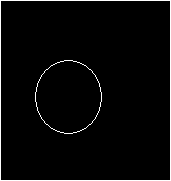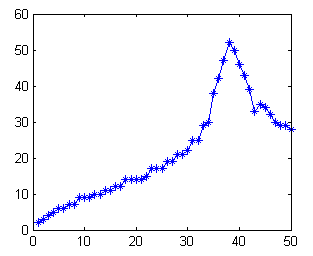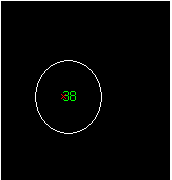### Hough Transform For Circle Detection - with unknown radius (I)

In previous 2 examples, the generalized Hough Transform has been applied to find for the circle with known radius. We were using the circle equation --> (x-a)^2 + (y-b)^2 = R^2 to map the pixel to a-b domain.

For detection circle with unknown radius, another parameter need to be make as variable, which is, the radius R.

Let's see how to perform this:

1. Reading image and the convert to binary image. After that, the location of the value '1' is found using 'find' function. (assume the image is already preprocess, if not, the 'edge' function might help)

clear all;clc;
I =im2bw(I);
[y,x]=find(I);
[sy,sx]=size(I);
imshow(I);2. Find all the require information for the transformatin. the 'totalpix' is the numbers of '1' in the image.

totalpix = length(x);

3. Preallocate memory for the Hough Matrix. The R is known in the range from 1 to 50, so we reserve 50 "layers" for the HM matrix.

HM = zeros(sy,sx,50);
R = 1:50;
R2 = R.^2;
sz = sy*sx;

4. Performing Hough Transform. Notice the accumulator is located in the inner for loop.

for cnt = 1:totalpix
for cntR = 1:50
b = 1:sy;
a = (round(x(cnt) - sqrt(R2(cntR) - (y(cnt) - [1:sy]).^2)));
b = b(imag(a)==0 & a>0);
a = a(imag(a)==0 & a>0);
ind = sub2ind([sy,sx],b,a);
HM(sz*(cntR-1)+ind) = HM(sz*(cntR-1)+ind) + 1;
end
end

5. Find for the maximum value for each layer, or in other words, the layer with maximum value will indicate the correspond R for the circle.

for cnt = 1:50
H(cnt) = max(max(HM(:,:,cnt)));
end
plot(H,'*-');6. Extract the information from the layer with maximum value, and overlap with the original image.

[maxval, maxind] = max(H);
[B,A] = find(HM(:,:,maxind)==maxval);
imshow(I); hold on;
plot(mean(A),mean(B),'xr')
text(mean(A),mean(B),num2str(maxind),'color','green')7. This example use the "for loop" extensively, and the speed of the program is rather slow. Vectorized the code might speed up the execution time, we wee see it in the next example. :)